geom_flow receives a dataset of the horizontal (x) and vertical (y, ymin, ymax) positions of the lodes of an alluvial plot, the intersections of the alluvia with the strata. It reconfigures these into alluvial segments connecting pairs of corresponding lodes in adjacent strata and plots filled x-splines between each such pair, using a provided knot position parameter knot.pos, and filled rectangles at either end, using a provided width.

geom_flow(
mapping = NULL,
data = NULL,
stat = "flow",
position = "identity",
width = 1/3,
knot.pos = 1/4,
knot.prop = TRUE,
curve_type = NULL,
curve_range = NULL,
segments = NULL,
aes.flow = "forward",
na.rm = FALSE,
show.legend = NA,
inherit.aes = TRUE,
...
)

positions_to_flow(
x0,
x1,
ymin0,
ymax0,
ymin1,
ymax1,
kp0,
kp1,
knot.prop,
curve_type,
curve_range,
segments
)

## Arguments

mapping

Set of aesthetic mappings created by aes(). If specified and inherit.aes = TRUE (the default), it is combined with the default mapping at the top level of the plot. You must supply mapping if there is no plot mapping.

data

The data to be displayed in this layer. There are three options:

If NULL, the default, the data is inherited from the plot data as specified in the call to ggplot().

A data.frame, or other object, will override the plot data. All objects will be fortified to produce a data frame. See fortify() for which variables will be created.

A function will be called with a single argument, the plot data. The return value must be a data.frame, and will be used as the layer data. A function can be created from a formula (e.g. ~ head(.x, 10)).

stat

The statistical transformation to use on the data; override the default.

position

Position adjustment, either as a string naming the adjustment (e.g. "jitter" to use position_jitter), or the result of a call to a position adjustment function. Use the latter if you need to change the settings of the adjustment.

width

Numeric; the width of each stratum, as a proportion of the distance between axes. Defaults to 1/3.

knot.pos

The horizontal distance of x-spline knots from each stratum (width/2 from its axis), either (if knot.prop = TRUE, the default) as a proportion of the length of the x-spline, i.e. of the gap between adjacent strata, or (if knot.prop = FALSE) on the scale of the x direction.

knot.prop

Logical; whether to interpret knot.pos as a proportion of the length of each flow (the default), rather than on the x scale.

curve_type

Character; the type of curve used to produce flows. Defaults to "xspline" and can be alternatively set to one of "linear", "cubic", "quintic", "sine", "arctangent", and "sigmoid". "xspline" produces approximation splines using 4 points per curve; the alternatives produce interpolation splines between points along the graphs of functions of the associated type. See the Curves section.

curve_range

For alternative curve_types based on asymptotic functions, the value along the asymptote at which to truncate the function to obtain the shape that will be scaled to fit between strata. See the Curves section.

segments

The number of segments to be used in drawing each alternative curve (each curved boundary of each flow). If less than 3, will be silently changed to 3.

aes.flow

Character; how inter-lode flows assume aesthetics from lodes. Options are "forward" and "backward".

na.rm

Logical: if FALSE, the default, NA lodes are not included; if TRUE, NA lodes constitute a separate category, plotted in grey (regardless of the color scheme).

show.legend

logical. Should this layer be included in the legends? NA, the default, includes if any aesthetics are mapped. FALSE never includes, and TRUE always includes. It can also be a named logical vector to finely select the aesthetics to display.

inherit.aes

If FALSE, overrides the default aesthetics, rather than combining with them. This is most useful for helper functions that define both data and aesthetics and shouldn't inherit behaviour from the default plot specification, e.g. borders().

...

Additional arguments passed to ggplot2::layer().

x0, x1, ymin0, ymax0, ymin1, ymax1, kp0, kp1

Numeric corner and knot position data for the ribbon of a single flow.

## Details

The helper function positions_toflow() takes the corner and knot positions and curve parameters for a single flow as input and returns a data frame of x, y, and shape used by grid::xsplineGrob() to render the flow.

## Aesthetics

geom_alluvium, geom_flow, geom_lode, and geom_stratum understand the following aesthetics (required aesthetics are in bold):

• x

• y

• ymin

• ymax

• alpha

• colour

• fill

• linetype

• size

• group

group is used internally; arguments are ignored.

## Curves

By default, geom_alluvium() and geom_flow() render flows between lodes as filled regions between parallel x-splines. These graphical elements, generated using grid::xsplineGrob(), are parameterized by the relative location of the knot (knot.pos). They are quick to render and clear to read, but users may prefer plots that use differently-shaped ribbons.

A variety of such options are documented at, e.g., this easing functions cheat sheet and this blog post by Jeffrey Shaffer. Easing functions are not (yet) used in ggalluvial, but several alternative curves are available. Each is encoded as a continuous, increasing, bijective function from the unit interval $$[0,1]$$ to itself, and each is rescaled so that its endpoints meet the corresponding lodes. They are rendered piecewise-linearly, by default using segments = 48. Summon each curve type by passing one of the following strings to curve_type:

• "linear": $$f(x)=x$$, the unique degree-1 polynomial that takes 0 to 0 and 1 to 1

• "cubic": $$f(x)=3x^{2}-2x^{3}$$, the unique degree-3 polynomial that also is flat at both endpoints

• "quintic": $$f(x)=10x^{3}-15x^{4}+6x^{5}$$, the unique degree-5 polynomial that also has zero curvature at both endpoints

• "sine": the unique sinusoidal function that is flat at both endpoints

• "arctangent": the inverse tangent function, scaled and re-centered to the unit interval from the interval centered at zero with radius curve_range

• "sigmoid": the sigmoid function, scaled and re-centered to the unit interval from the interval centered at zero with radius curve_range

Only the (default) "xspline" option uses the knot.* parameters, while only the alternative curves use the segments parameter, and only "arctangent" and "sigmoid" use the curve_range parameter. (Both are ignored if not needed.) Larger values of curve_range result in greater compression and steeper slopes. The NULL default will be changed to 2+sqrt(3) for "arctangent" and to 6 for "sigmoid".

These package-specific options set global values for curve_type, curve_range, and segments that will be defaulted to when not manually set:

• ggalluvial.curve_type: defaults to "xspline".

• ggalluvial.curve_range: defaults to NA, which triggers the curve-specific default values.

• ggalluvial.segments: defaults to 48L.

See base::options() for how to use options.

## Defunct parameters

The previously defunct parameters axis_width and ribbon_bend have been discontinued. Use width and knot.pos instead.

ggplot2::layer() for additional arguments and stat_alluvium() and stat_flow() for the corresponding stats.

Other alluvial geom layers: geom_alluvium(), geom_lode(), geom_stratum()

## Examples

# use of strata and labels
ggplot(as.data.frame(Titanic),
aes(y = Freq,
axis1 = Class, axis2 = Sex, axis3 = Age)) +
geom_flow() +
scale_x_discrete(limits = c("Class", "Sex", "Age")) +
geom_stratum() +
geom_text(stat = "stratum", aes(label = after_stat(stratum))) +
ggtitle("Alluvial plot of Titanic passenger demographic data")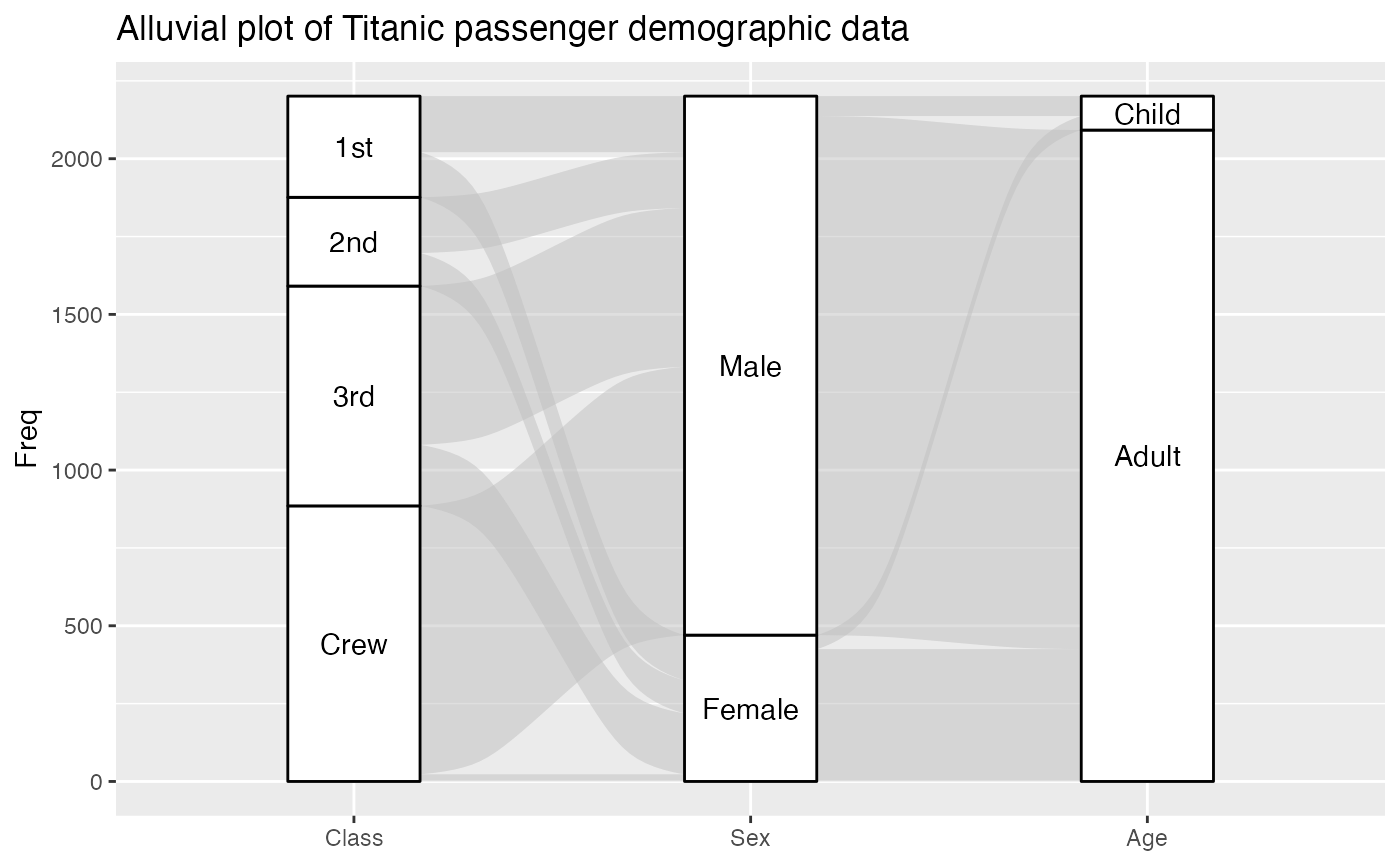# use of facets, with sigmoid flows
ggplot(as.data.frame(Titanic),
aes(y = Freq,
axis1 = Class, axis2 = Sex)) +
geom_flow(aes(fill = Age), width = .4, curve_type = "quintic") +
geom_stratum(width = .4) +
geom_text(stat = "stratum", aes(label = after_stat(stratum)), size = 3) +
scale_x_discrete(limits = c("Class", "Sex")) +
facet_wrap(~ Survived, scales = "fixed")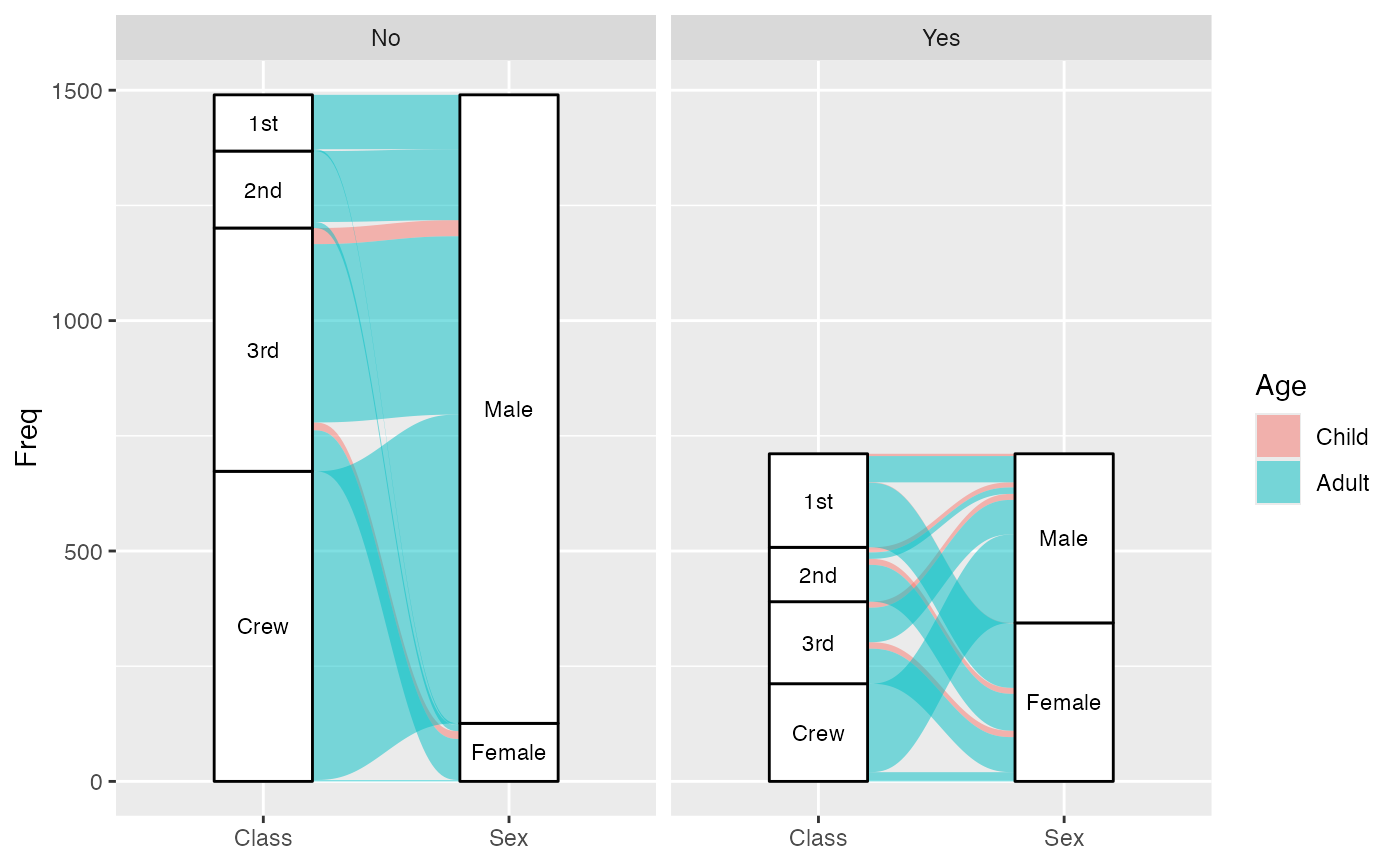# time series alluvia of WorldPhones data
wph <- as.data.frame(as.table(WorldPhones))
names(wph) <- c("Year", "Region", "Telephones")
ggplot(wph,
aes(x = Year, alluvium = Region, y = Telephones)) +
geom_flow(aes(fill = Region, colour = Region), width = 0)# treat 'Year' as a number rather than as a factor
wph$Year <- as.integer(as.character(wph$Year))
ggplot(wph,
aes(x = Year, alluvium = Region, y = Telephones)) +
geom_flow(aes(fill = Region, colour = Region), width = 0)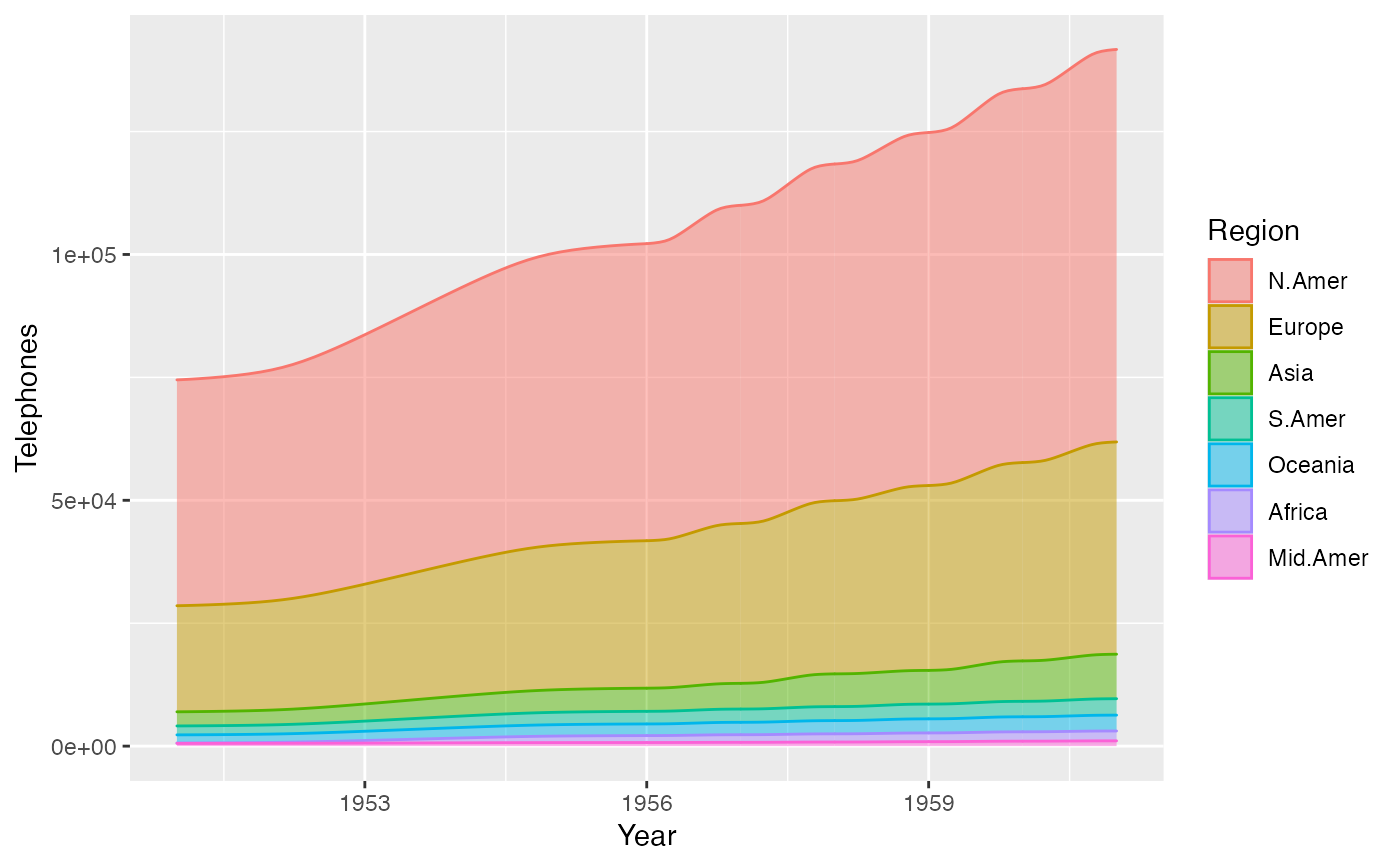# hold the knot positions fixed
ggplot(wph,
aes(x = Year, alluvium = Region, y = Telephones)) +
geom_flow(aes(fill = Region, colour = Region), width = 0, knot.prop = FALSE)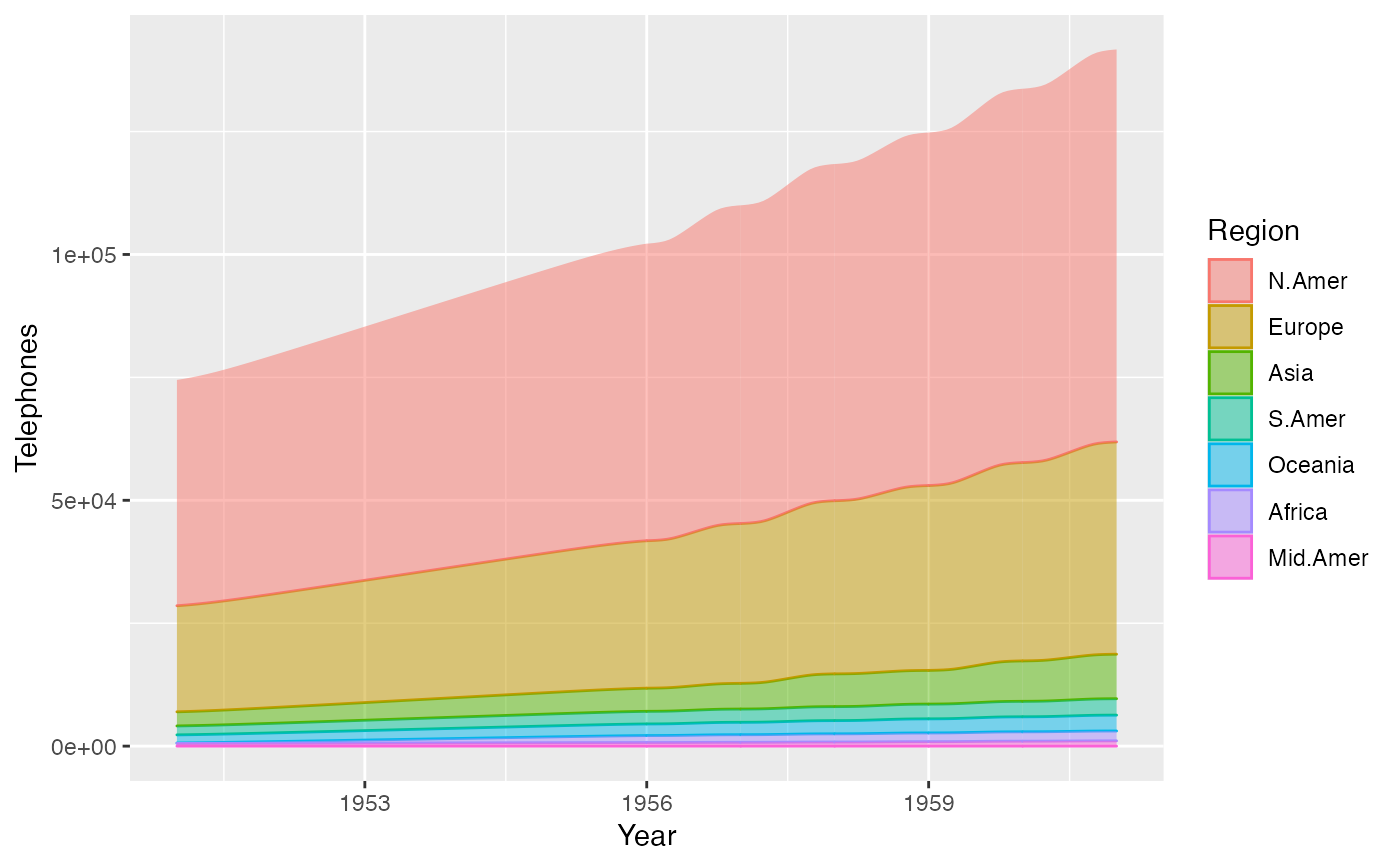# \donttest{
# rightward flow aesthetics for vaccine survey data, with cubic flows
data(vaccinations)
vaccinations$response <- factor(vaccinations$response,
rev(levels(vaccinations\$response)))
# annotate with proportional counts
ggplot(vaccinations,
aes(x = survey, stratum = response, alluvium = subject,
y = freq, fill = response)) +
geom_lode() + geom_flow(curve_type = "cubic") +
geom_stratum(alpha = 0) +
geom_text(stat = "stratum", aes(label = round(after_stat(prop), 3)))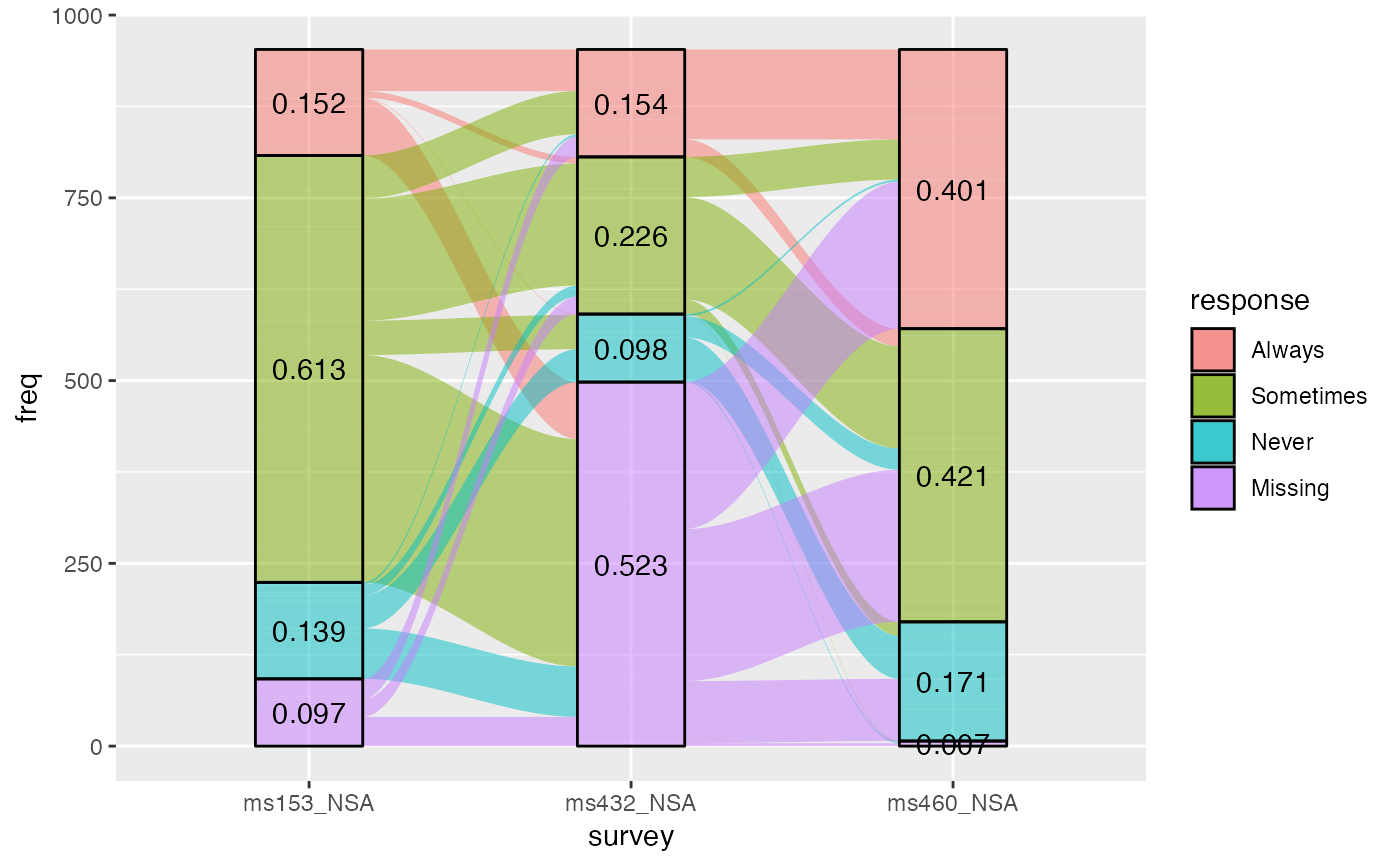# annotate fixed-width ribbons with counts
ggplot(vaccinations,
aes(x = survey, stratum = response, alluvium = subject,
weight = freq, fill = response)) +
geom_lode() + geom_flow(curve_type = "cubic") +
geom_stratum(alpha = 0) +
geom_text(stat = "flow",
aes(label = after_stat(n),
hjust = (after_stat(flow) == "to")))# }